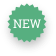# The Elasticity of Demand for Gasoline

Published 21 Aug 2015
Reference 6159
Topic
Industry
Region
Length 5 page(s)
Summary

This exercise uses monthly data for the US from 1978-2013 to estimate a demand function for gasoline in the US. The demand function is used to calculate the price and income elasticity of demand. Subsequently, it shows how to estimate elasticities in the short and the long run.

Teaching objectives

First, to teach the concept of elasticity by showing that elasticity of demand can be estimated using data and regression analyses. Second, to use these concepts to explain why oil prices tend to be so volatile in the short run. Students use the data and built-in regression functions in Excel (file available on request) to estimate elasticity of demand for gasoline. Next, they estimate short and long-run elasticities to see that demand is much more price-inelastic in the short run. The exercise is useful for both MBA students and for students in an intermediate Microeconomics course.

Keywords
• Q41415

## Recently Viewed